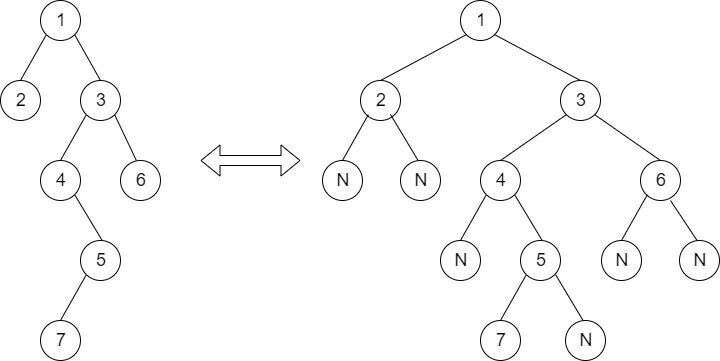Deletion in a Binary Tree
Easy Accuracy: 35.27% Submissions: 10824 Points: 2

Given a Binary Tree of size N, your task is to complete the function deletionBT(), that should delete a given node from the tree by making sure that tree shrinks from the bottom (the deleted node is replaced by bottommost and rightmost node).
Example:

Input:
First line of input contains the number of test cases T. For each test case, there will be two lines of input , First line contains given node value and second line is a string representing the tree as described below:

1. The values in the string are in the order of level order traversal of the tree where, numbers denote node values, and a character “N” denotes NULL child.

2. For example:For the above tree, the string will be: 1 2 3 N N 4 6 N 5 N N 7 N

Output:
For each test output will be the inorder traversal of the new tree.
You don't have to take input. Complete the function deletionBT() that takes root node of the tree and given node value as parameter and modifies the tree.
Constraints:
1<=T<=30
1<=N<=104
Example:
Input:

2
1
1 4 7 5 6
5
7 10 4 11 2 5 9
Output:
5 4 6 7
11 10 2 7 9 4

Note:The Input/Ouput format and Example given are used for system's internal purpose, and should be used by a user for Expected Output only. As it is a function problem, hence a user should not read any input from stdin/console. The task is to complete the function specified, and not to write the full code.